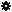﻿ Object Model: NurbsSurface.EvaluateNormalizedPosition

NurbsSurface.EvaluateNormalizedPosition

## NurbsSurface.EvaluateNormalizedPosition### Description

Returns the Position and optionally U tangent, V Tangent,Normal at a given normalized UV value.

Note: This method uses output arguments. C# and some scripting languages (such as JScript and PerlScript) don't support arguments passed by reference. However, there is a alternate version of this method which is considered safe to use with C#, JScript and PerlScript: NurbsSurface.EvaluateNormalizedPosition2.

### C# Syntax

 ```NurbsSurface.EvaluateNormalizedPosition( Double in_dUValueNormalized, Double in_dVValueNormalized, Object& out_pPosition, Object& out_pUTangent, Object& out_pVTangent, Object& out_pNormal ); ```

### Scripting Syntax

 ```NurbsSurface.EvaluateNormalizedPosition( UValueNormalized, VValueNormalized, [Position], [UTangent], [VTangent], [Normal] ); ```

### Parameters

Parameter Type Description
UValueNormalized Double The normalized UValue(0.0 to 1.0) at which we want to evaluate the surface.
VValueNormalized Double The normalized VValue(0.0 to 1.0) at which we want to evaluate the surface.
Position SIVector3 (contains the position) Position at the given UV.
UTangent SIVector3 (contains the U tangent) Tangent in U at the given UV.
VTangent SIVector3 (contains the V tangent) Tangent in V at the given UV.
Normal SIVector3 (contains the normal) Normal at the given UV.

### Examples

#### VBScript Example

 ```set oRoot = Application.ActiveProject.ActiveScene.Root set oSphere = oRoot.AddGeometry( "Sphere", "NurbsSurface" ) oSphere.ActivePrimitive.Geometry.Surfaces(0).EvaluateNormalizedPosition _ 0.5, 0.5, oPosition,oUTangent,oUTangent,oNormal LogMessage "The position at 0.5, 0.5 is x :" & oPosition.x & " y: " & _ oPosition.y & " z: " & oPosition.z ```